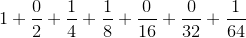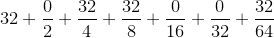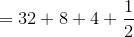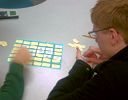Search IntMath
Close

# IntMath Newsletter: Binary scientific notation, math game

By Murray Bourne, 31 Aug 2015

31 Aug 2015

1. Binary numbers in scientific notation
2. Math game: Antiderivative Block
3. Math puzzles
4. Math movie: Math is forever
5. Final thought: When the last fish...

You may have noticed the frequency of Newsletters has dropped lately. This is normal over the Northern hemisphere summer period, but also I've been very busy with multiple projects. Thank you for your patience!

## 1. Binary numbers in scientific notation

Binary numbers are interesting because they're used in computers and many other electronic devices (since they represent 1 = "on" and 0 = "off").

Ever wondered how to write very small (or large) binary numbers?

First, a reminder.

### Scientific notation - decimal numbers

As we learned on the Scientific Notation page, we write the (large-ish) number 472,800 in scientific notation as follows:

4.728 × 105

The digits in our number are in decreasing powers of 10:

4.728 = 4 × 100 + 7 × 10−1 + 2 × 10−2 + 8 × 10−3

Multiplying each of those terms by 105 gives us:

4.723 × 105 = 4 × 105 + 7 × 104 + 2 × 103 + 8 × 102 = 472,800.

### Scientific notation - binary numbers

In the binary system, we work in base 2, and we can only use two digits, 0 and 1.

An example of a binary number in scientific notation looks like this: 1.011001 × 25. (Yes, the number and power at the end are written in base 10 numbers.) The value of this number is:

1.011001 × 25

= (1 × 20 + 0 × 2−1 + 1 × 2−2 + 1 × 2−3 + 0 × 2−4 + 0 × 2−5 + 1 × 2−6) × 25.

Multiplying the part in brackets by 25 gives:

1 × 25 + 0 × 24 + 1 × 23 + 1 × 22 + 0 × 21 + 0 × 20 + 1 × 2−1

= 3210 + 810 + 410 + 1/2 = 44.510

(I've used the subscript "10" to indicate they are base 10 numbers.)

### As fractions

In fractions, the part in brackets is equivalent to:Multiplying each term by 25 = 32 gives us:= 44.510

### Floating point numbers aren't real

The above concept is relevant to the problem I mentioned in the last Newsletter, about YouTube's large number issue involving Psy's Gangnam Style video.

I came across an interesting related article recently by Chuck Allison, in the series 97 Things Every Programmer Should Know.

In the article Floating Point Numbers Aren't Real, we learn that:

IEEE floating-point numbers are fixed-precision numbers based on base-two scientific notation: 1.d1d2...dp−1 × 2e

where p is the precision (24 for float or 32-bit, 53 for double, or 64-bit). You can see my example above is in this format.

The article explains why there is a problem with very large (and very small) numbers on computers ("floating-point numbers are approximations of real numbers"), and how precision of the number affects the outcome.

The author gives some warnings about coding without being aware of the limitations of floating point numbers, especially when financial matters are involved.

## 2. Resource: Antiderivative Block math game

Many math classes are really dry, but it doesn't have to be that way. There are plenty of good "serious" math games that can help students learn math in novel ways.

Maria H. Andersen of Teaching College Math (now Busynessgirl) has developed and collected an interesting set of math games. Many of them are low-tech (paper-based), while others are for tablets or other mobile devices.In Antiderivative Block, students are encouraged to "(1) learn their derivative rules well (2) begin thinking about derivatives backwards, and (3) to learn to be careful not to mix up derivatives and antiderivatives".

There's also:

## 3. Math puzzles

Correct answers with explanation were given by: Paul G, Don Miller, Henry, Tom Barrett, Rutwink Tank, and Toma Garza. Some of these solutions included a check step, which is always a good idea.

Some of the other responses included the answer only. I've had several students during my teaching career that just "see" a solution, and have trouble explaining how they derived it. I think that's perfectly reasonable, except:

1. It doesn't help other people learn how to find the answer.
2. Such an approach often cannot be generalised for similar, but more difficult questions (or ones where the numbers are not "nice").

### New math puzzle

There are cards numbered 1, 2, 3, ... , 2000 face down on a table. If I choose one at random, what is the probability the number on it will contain a 5?

Bonus point: If the cards use Roman numerals instead, what is the probability the card we choose contains a "V"?

You can leave your responses here.

## 4. Math movie - de Cabezón: Math is forever

The opening lines of the video:

Imagine you're in a bar, or a club,
and you start talking, and after a while, the question comes up,
"So, what do you do for work?"
And since you think your job is interesting,
you say, "I'm a mathematician."Eduardo Sáenz de Cabezón s discusses the utility of mathematics in this interesting TED talk. It's in Spanish, with English subtitles.

## 5. Final thought: When the last fish...

First Nations Canadian filmmaker and singer-songwriter Alanis Obomsawin has a great quote about money.

When the last tree is cut, the last fish is caught, and the last river is polluted; when to breathe the air is sickening, you will realize, too late, that wealth is not in bank accounts and that you can’t eat money. [Alanis Obomsawin]

Until next time, enjoy whatever you learn.

### Comment Preview

HTML: You can use simple tags like <b>, <a href="...">, etc.

To enter math, you can can either:

1. Use simple calculator-like input in the following format (surround your math in backticks, or qq on tablet or phone):
a^2 = sqrt(b^2 + c^2)
(See more on ASCIIMath syntax); or
2. Use simple LaTeX in the following format. Surround your math with $$ and $$.
$$\int g dx = \sqrt{\frac{a}{b}}$$
(This is standard simple LaTeX.)

NOTE: You can mix both types of math entry in your comment.